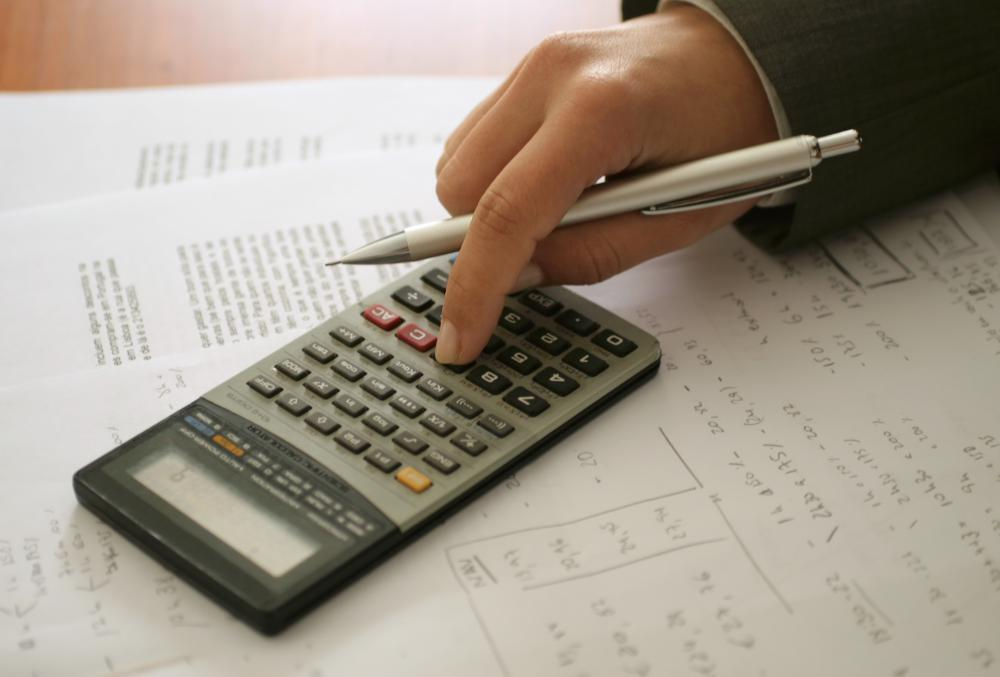# What is an Empirical Probability?

Leo Zimmermann

Empirical probability is a calculation of likelihood based on the actual occurrence of a certain type of event. It is distinct from estimated, or theoretical, probability, which produces a value based on general principles rather than observed fact. Empirical probability describes a more inductive process, one which decreases error resulting from incorrect models but increases error resulting from random events.Empirical probability is a calculation of likelihood based on the actual occurrence of a certain type of event.

A simple example for understanding the two types of probabilities is a simple repeated coin flip. Say a coin is flipped 100 times. It comes up heads 54 times and tails 46 times. There are two different ways to estimate the probability that the next toss will come up heads. The theoretical probability is 50 percent. This probability remains constant from flip to flip. The empirical probability, on the other hand, is 54%. The coin has come up heads 54% of the time so far; based only on this data, one might expect that it is slightly more likely to come up heads again. The empirical probability changes with the arrival of new data. If after 200 flips, the coin has come up heads 104 times, the empirical probability of the next coin being heads is now 52%.

Empirical probabilities become more trustworthy the more data there is. If the model for producing the theoretical probability is good—in the above example, if the coin is fair—the theoretical and empirical probabilities will converge as the sample size grows larger. After a million coin flips, an observer should expect the empirical probability to be very close to the predicted probability, 50%.

The more the two types of probability diverge, the more an observer might consider changing the parameters of his or her model for theoretical probability. In the classic gambler's fallacy, in which a coin comes up heads 99 times, a basic math textbook will say that the next coin still has a 50% chance of being tails. This answer is based on the assumption that the coin is fair: that it has evenly distributed weight and air resistance, that it is tossed effectively and randomly, and so on. The estimated probability might tell the gambler in this situation that the coin is not fair. Extreme deviance from the theoretical probability suggests that there may be something wrong with one of the assumptions used to calculate it.

Empirical probability does not always have to be the double of theoretical probability. It might be used to calculate the probability of an event about which little else is known. For example, if a person were flipping a two sided object whose two sides have different properties, she might rely more heavily on an empirical element of the probability of it landing on a certain side. Once again, the more data she has, the higher the quality of her empirical calculation.

People in the fields of economics and finance might well use empirical probability to help inform their decisions. An economist, after creating a theoretical model of a market, should want to check her calculations against an empirical calculation of the probabilities involved. She might rely heavily on empirical probabilities to fill coefficients in her model that she might have no other way of calculating. In practice, useful economic models almost always combine elements of theoretical and empirical probability.

## You might also LikeSteamLouis

I think that empirical probability is more accurate if the data is considered alongside results. For example, we can calculate the probability of stocks losing value in one sector using empirical probability. If stocks in that sector have lost value very often lately, than we know that empirical probability should give similar results.

bear78

@bluedolphin-- You're absolutely right.

A large sample size is required for empirical probability results to be very accurate. That's why alternative statistical models are recommended. If there are alternative estimates of a probability, then the risk of encountering a fallacy becomes lower. Other statistical models can also indicate the accuracy of the empirical probability results.

Empirical probability may not be the most accurate, but it is still preferred in some cases because it's based on real events/data. It's advantageous that the probability doesn't have to rely on assumptions.

bluedolphin

So fallacies are common with empirical probability?

It sounds like, in order to get an accurate probability, the sample size needs to be very, very large. And that's not always possible. Who can flip a coin a million times?

Why is this calculation used when it's not very accurate?